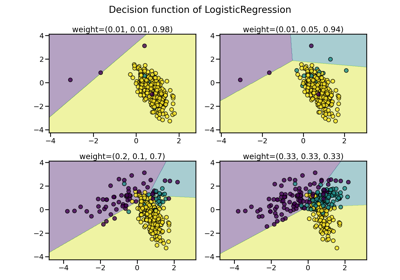# imblearn.over_sampling.KMeansSMOTE¶

class imblearn.over_sampling.KMeansSMOTE(*, sampling_strategy='auto', random_state=None, k_neighbors=2, n_jobs=None, kmeans_estimator=None, cluster_balance_threshold='auto', density_exponent='auto')[source]

Apply a KMeans clustering before to over-sample using SMOTE.

This is an implementation of the algorithm described in .

Read more in the User Guide.

Parameters
sampling_strategyfloat, str, dict or callable, default=’auto’

Sampling information to resample the data set.

• When float, it corresponds to the desired ratio of the number of samples in the minority class over the number of samples in the majority class after resampling. Therefore, the ratio is expressed aswhereis the number of samples in the minority class after resampling andis the number of samples in the majority class.

Warning

float is only available for binary classification. An error is raised for multi-class classification.

• When str, specify the class targeted by the resampling. The number of samples in the different classes will be equalized. Possible choices are:

'minority': resample only the minority class;

'not minority': resample all classes but the minority class;

'not majority': resample all classes but the majority class;

'all': resample all classes;

'auto': equivalent to 'not majority'.

• When dict, the keys correspond to the targeted classes. The values correspond to the desired number of samples for each targeted class.

• When callable, function taking y and returns a dict. The keys correspond to the targeted classes. The values correspond to the desired number of samples for each class.

random_stateint, RandomState instance, default=None

Control the randomization of the algorithm.

• If int, random_state is the seed used by the random number generator;

• If RandomState instance, random_state is the random number generator;

• If None, the random number generator is the RandomState instance used by np.random.

k_neighborsint or object, default=2

If int, number of nearest neighbours to used to construct synthetic samples. If object, an estimator that inherits from sklearn.neighbors.base.KNeighborsMixin that will be used to find the k_neighbors.

n_jobsint, default=None

Number of CPU cores used during the cross-validation loop. None means 1 unless in a joblib.parallel_backend context. -1 means using all processors. See Glossary for more details.

kmeans_estimatorint or object, default=None

A KMeans instance or the number of clusters to be used. By default, we used a sklearn.cluster.MiniBatchKMeans which tend to be better with large number of samples.

cluster_balance_threshold“auto” or float, default=”auto”

The threshold at which a cluster is called balanced and where samples of the class selected for SMOTE will be oversampled. If “auto”, this will be determined by the ratio for each class, or it can be set manually.

density_exponent“auto” or float, default=”auto”

This exponent is used to determine the density of a cluster. Leaving this to “auto” will use a feature-length based exponent.

SMOTE

Over-sample using SMOTE.

SVMSMOTE

Over-sample using SVM-SMOTE variant.

BorderlineSMOTE

Over-sample using Borderline-SMOTE variant.

ADASYN

References

1

Felix Last, Georgios Douzas, Fernando Bacao, “Oversampling for Imbalanced Learning Based on K-Means and SMOTE” https://arxiv.org/abs/1711.00837

Examples

>>> import numpy as np
>>> from imblearn.over_sampling import KMeansSMOTE
>>> from sklearn.datasets import make_blobs
>>> blobs = [100, 800, 100]
>>> X, y  = make_blobs(blobs, centers=[(-10, 0), (0,0), (10, 0)])
>>> # Add a single 0 sample in the middle blob
>>> X = np.concatenate([X, [[0, 0]]])
>>> y = np.append(y, 0)
>>> # Make this a binary classification problem
>>> y = y == 1
>>> sm = KMeansSMOTE(random_state=42)
>>> X_res, y_res = sm.fit_resample(X, y)
>>> # Find the number of new samples in the middle blob
>>> n_res_in_middle = ((X_res[:, 0] > -5) & (X_res[:, 0] < 5)).sum()
>>> print("Samples in the middle blob: %s" % n_res_in_middle)
Samples in the middle blob: 801
>>> print("Middle blob unchanged: %s" % (n_res_in_middle == blobs + 1))
Middle blob unchanged: True
>>> print("More 0 samples: %s" % ((y_res == 0).sum() > (y == 0).sum()))
More 0 samples: True

Attributes
kmeans_estimator_estimator

The fitted clustering method used before to apply SMOTE.

nn_k_estimator

The fitted k-NN estimator used in SMOTE.

cluster_balance_threshold_float

The threshold used during fit for calling a cluster balanced.

__init__(self, *, sampling_strategy='auto', random_state=None, k_neighbors=2, n_jobs=None, kmeans_estimator=None, cluster_balance_threshold='auto', density_exponent='auto')[source]

Initialize self. See help(type(self)) for accurate signature.

## Examples using imblearn.over_sampling.KMeansSMOTE¶